# O Level A Math Tuition(Improve 2 Grades Or More)(Express/IP/NA)

## Are you worried that your child is not doing well in A Math?

A Math Tuition, is it really needed? In Odyssey Math, we understand students’ frustrations when it comes to learning Additional Mathematics, especially when they struggle in the subject when school teachers go through the chapters too quickly and are unable to attend to students’ queries due to large class size. As such, they are unable to catch up with the syllabus and more often than not, due to the lack of understanding of the topics, their grades suffer and they continue to do badly. In Odyssey Math, we aim to provide high quality math tuition by delivering lessons in a simplified approach, allowing every student to understand A Math and realise their fullest potential. As such, they will be able to make improvement in the subject.

### \$240/ 4 weeks (Sec)\$280/ 4 weeks (JC)

Affordable, no hidden charges, no registration and administrative fees.

### Free Trial Available

First lesson is a free trial! No obligations to continue!

### Free Math Videos, over 800+

Free online math videos (see below!) for our enrolled students, totally free.

### Math Tuition

Small class size of 6 to 8, no such thing as large classes.

We ensure that even after tuition, students still get their questions answered.

## Math tuition that provides FREE e-learning for enrolled students

0 +
Hours of Development, Specially Curated For Our Math Students
0
Topical Videos and Practice Questions For Additional Math
0
Topical Videos and Practice Questions For Elementary Math
0
Topical Videos For H2 Math

## Why Odyssey Math?

### Results Oriented

Parents send their children to math tuition hoping to see an improvement in their math results. If not, why bother sending their children to tuition in the first place? Here in Odyssey Math, Mr Tan’s vast resources, including both physical and online materials will allow students to not only accelerate their learning but also allow them to recap A Math concepts whenever they need to. In addition to physical lessons, enrolled students are able to watch online math videos on our website to recap concepts that they forgot or are weak in. With a strategic approach in helping students do well in A Math as well as topical tests to see if student understood the chapters that Mr Tan has gone through, Mr Tan believes that if students follow his guidance and make use of his resources, they will be able to do well in A Math.

### Experienced and Qualified Math Tutor

11 years of coaching mathematics and counting, Mr Tan knows it all, from explaining A Math concepts simply to providing tips and tricks to solve questions as well as knowing where students will tend to make mistakes. He is able to provide the best guidance to aid students in scoring well for A Math. Ultimately, it is Mr Tan’s love and passion for Mathematics as well as imparting knowledge to his students that pushes him to deliver his best whenever students come for his lessons. Graduated in 2018 from NUS with a distinction in Applied Mathematics (Primary Major) with a secondary major in economics, he hopes to provide the best math tuition for his students.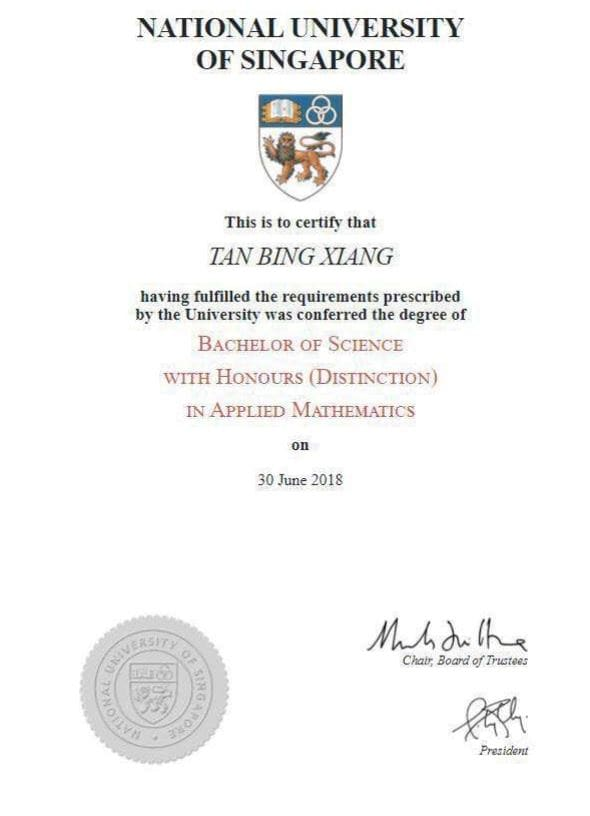### Affordable A Math Tuition with maximum value

With rising tuition costs particularly 1 to 1 tuition in Singapore, it has become a burden to many parents. In Odyssey Math, Mr Tan understands this and seek to provide the highest quality math tuition and at the same time keep the tuition fees low. This is our mission to reduce the cost burden for parents. At the same time, we sincerely believe that with our dedication and passion towards teaching mathematics, our students are the one to benefit the most from our lessons

Mr Tan’s believes that education does not stop after lesson hours. If students have queries related to A Math, they can ask him via WhatsApp.

### Small Class Sizes

Parents are often worried about large class size. In Odyssey Math, there’s no such thing as large class size. Our class size is capped at 6 to 8 students so that every student can receive individualized attention.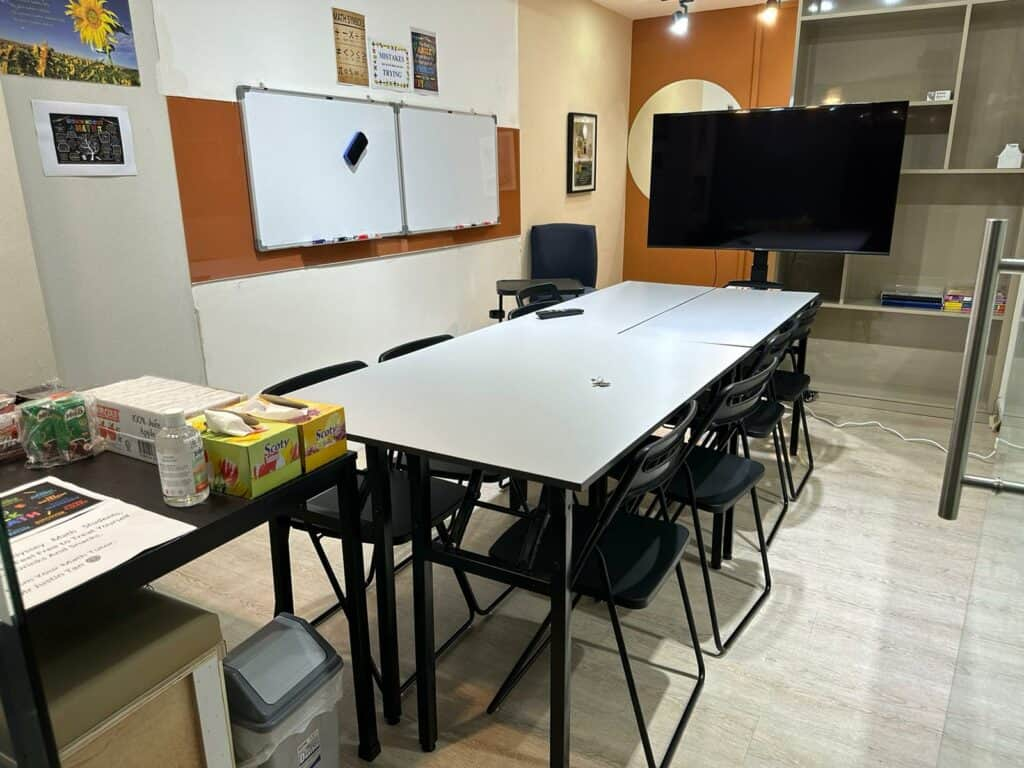### Testimonials of our teaching

Don’t take our words for it. Look at the testimonials of our students below to see what they have to say.

## Our A Math Tuition Structure

Begin with the end in mind, that’s how we approach getting things done. We will break down the learning into 4 phases, the first phase which is the introduction of topics. We explain concepts to students in a simplified manner after which we will provide them practice questions in class and resolve their misconceptions. Homework will be assigned and reviewed in subsequent lesson. Topical test will be given to students to test their understanding of the chapters after which we will review the concepts with them again. Through this systematic approach, we allow students to pace themselves well and understand mathematics better.

## A Math Topics Covered

• Using the method of completing the square to find the maximum or minimum value of a quadratic function
• Conditions for graph to be always positive (or always negative)
• Using quadratic functions as models
• Conditions for a quadratic equation to have two equal roots
• Conditions for a quadratic equation to have two real roots
• Conditions for a quadratic equation to have no real roots
• Related conditions for a given line to intersect a given curve
• Related conditions for a given line to be a tangent to a given curve
• Related conditions for a given line to not intersect a given curve
• Solve simultaneous equations in two variables by substitution, with one of the equations being non-linear equation
• Solving quadratic inequalities and representing the solution on the number line
• Operations on surds
• How to rationalize the denominator
• Know how to solve equations involving surds
• Multiplication and division of polynomials
• Factorizing polynomials, know how to use remainder and factor theorems
• Solve cubic equations
• Sum and differences of cubes
• Know how to split fractions where denominator can be linear factor, repeated linear factor or quadratic factor which cannot be factorised
• Know how to use Binomial Theorem for positive integer n
• Understanding of factorial and n choose r
• Understand the general term
• Laws of logarithm
• Exponential Function
• Logarithmic Function
• Change of base of logarithms
• Know how to simplify expressions and solve equations involving exponential and logarithmic functions
• Using exponential and logarithmic functions as models
• Know sine, cosine, tangent, secant, cosecant and cotangent (six trigonometric functions)
• Principle values of inverse trigonometric functions of sine, cosine and tangent
• Exact values of the trigonometric functions for special angles
• Amplitudes, periodicity and symmetries related to sine and cosine functions
• Graphs of sine, cosine and tangent
• Trigonometric Identities
• Double Angle Formulae
• R-Formula
• Know how to simplify trigonometric expressions
• Know how to find solution of trigonometric equations in a given interval (excluding general solution)
• Know how to prove simple trigonometric identities
• Usage of trigonometric functions as models
• Conditions for two lines to be parallel or perpendicular
• Midpoint of line segment
• Rectilinear figure area
• Know two forms for the equation of circle
• Linear Law, know how to transform equations to a linear form Y=mX+c
• Assumed knowledge of geometry properties in O Level Mathematics
• Assumed knowledge of Congruence and Similarity in O Level Mathematics
• Midpoint Theorem
• Alternate Segment Theorem (tangent chord theorem)
• Derivative of f(x)
• Rate of Change
• Use of standard notations for derivative
• Derivative of algebraic expressions, trigonometric functions, exponential functions and exponential functions with constant multiples, sums and differences
• Derivative of products and quotients of functions
• Power Rule
• Product Rule
• Quotient Rule
• Chain Rule
• Increasing and decreasing functions
• Stationary points
• Maximum and minimum turning points
• Stationary point of inflexion
• First derivative test
• Second derivative test
•  Tangents and Normals
• Connected Rate of Change
• Maxima and Minima Problems
• Understanding integration as the reverse of differentiation
• Integration of algebraic expressions, trigonometric functions, exponential functions and exponential functions with constant multiples, sums and differences
• Definite Integral relating to area under a curve
• Evaluating Definite Integral
• Finding the area of a region bounded by a curve and line(s) (excluding between two curves)
• Understand how to find area of region below the x-axis
• Kinematics through the use of differentiation and integration to solve problems involving displacement, velocity and acceleration of an object moving in a straight line

## What Our Students Have to Say about Odyssey Math Tuition

Mr Tan is very dedicated in teaching A and E Math. I am able to ask questions related to mathematics and will be able to get a reply asap. Notes and questions provided are scaled to the student's personal standards. My understanding of Additional and Elementary Math has made a lot of improvements under his teaching by being able to cope better with this subject.Vernasa
Mr Tan is a very approachable, friendly and patient tutor. His teachings are easily understandable, clear and helpful. Whenever i face problems or have difficulties, he will be there to guide me and ensure that i fully understand my doubts before continuing. The math questions he provided are unique and helpful in allowing me to understand the concepts better. His math tuition has allowed me to understand mathematics better.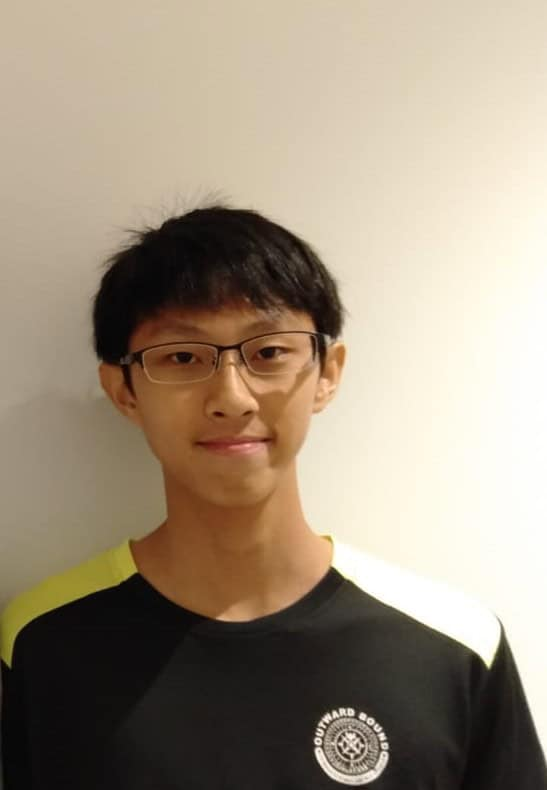Beyvlen Koh
In Mr Tan's math tuition, he go through his math lessons with a lot of details. I enjoyed the way he explain questions, especially when he use several methods to explain the solutions. He will provide alternative solutions for us to understand the questions better. The questions he provided allow me to have a good grasp of the math concepts. The pace of his explanation allows me to understand better. Thank you Mr Tan!Yun Tian Yi
Mr Tan is very knowledgeable in mathematics and he is very efficient in teaching his students. Even though i only spent a few sessions with him just before my O level, i have already learnt what i needed to learn based on the syllabus. I get to clarify my doubts and misconceptions in mathematics with him and he is able to answer me quickly. I would highly recommend everyone to try out his math tuition.Algernon Quek
The math questions provided are well thought-out and it is up to the standard for average students. After practicing the questions, Mr Tan will explain every questions patiently and make sure i understand not just the solutions but also the process of tackling the questions. He is always willing to answer any inquiries. Thank you so much Mr Tan!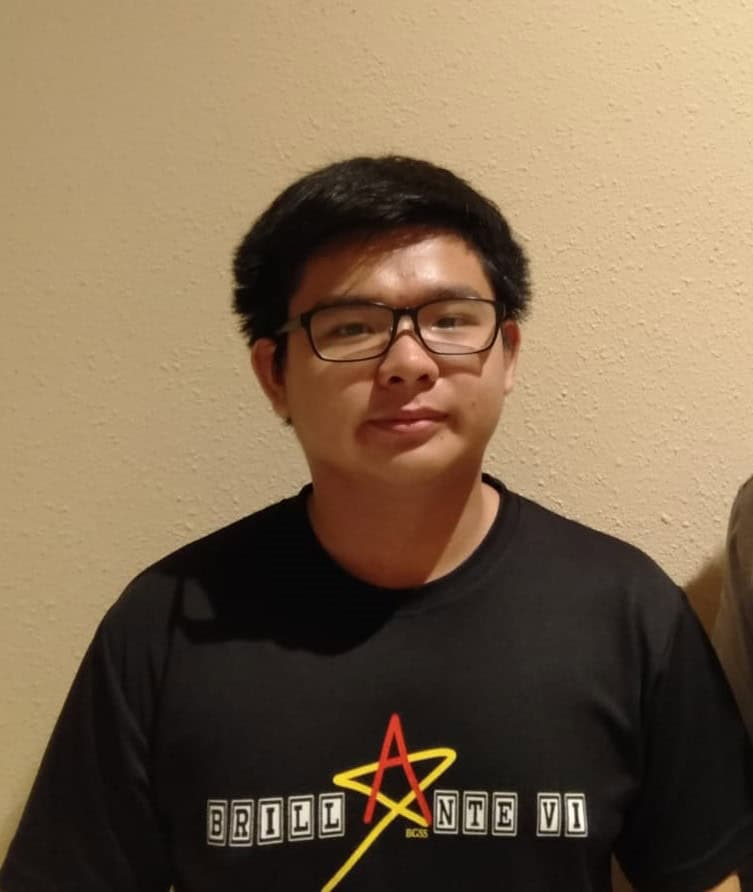Teo Yi Hao
Mr Tan's lessons were very productive and he will always put in the extra effort to teach us after the lesson which he is not oblige to do so. He would stay after the lessons to answer our queries and go through the math questions that we asked. His explanations are clear and simple. At the end of the explanation, he made me felt like the questions were actually quite easy to tackle. Thanks a lot Mr Tan and i appreciate your passion in teaching mathematics!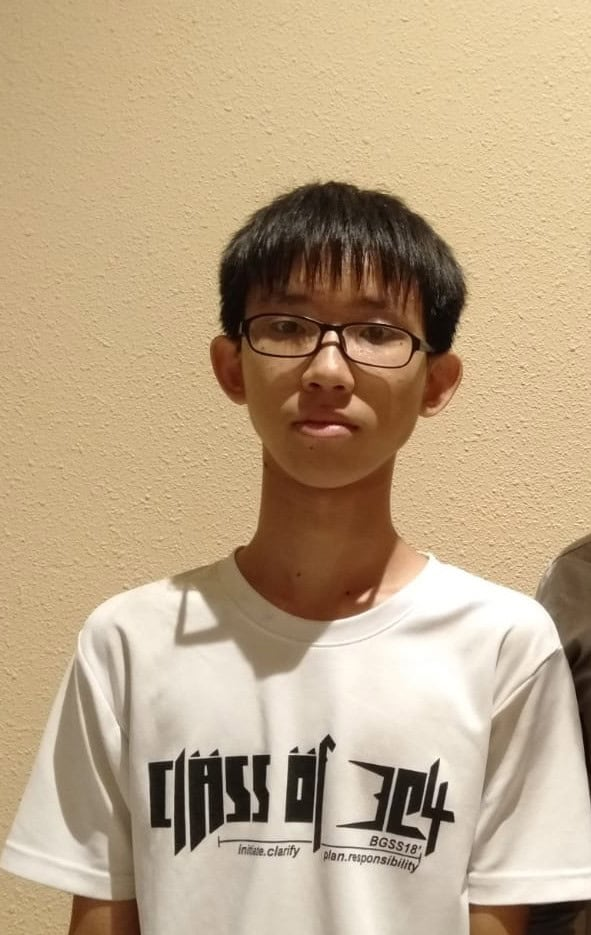Beyvlen Koh
Before going for Mr Tan's math tuition, i had a lot of doubts about the sessions. After going for his sessions, it was the best decision ever made. He is a really nice teacher who is patient in teaching. From my point of view, he teaches really well and his explanation is clear and concise. I could feel that our improvement in math grades will be his greatest achievement. He always does his best in teaching and make sure that we understand the concepts better. I would recommend students to join his lessons.